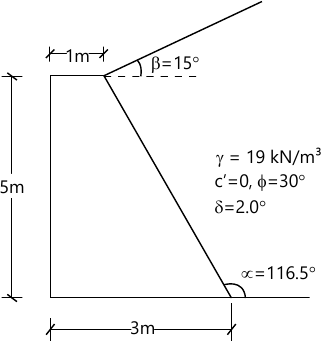MORE IN Geotechnical Engineering - 2
MU Civil Engineering (Semester 6)
Geotechnical Engineering - 2
May 2014
Total marks: --
Total time: --
INSTRUCTIONS
(1) Assume appropriate data and state your reasons
(2) Marks are given to the right of every question
(3) Draw neat diagrams wherever necessary

1 (a) Differentiate between earth pressure at rest, active and passive condition.
5 M
1 (b) What is a general local and punching shear failure of shallow foundation?
5 M
1 (c) Explain pile foundations on the basis of load transfer and method of installation.
5 M
1 (d) What are the different factor of safety used in the stability analysis of slopes.
5 M

2 (a) Explain the difference between Rankine's and Coulomb's earth pressure theories.
10 M
2 (b) A retaining wall 2m in height has a smooth vertical surface. The backfill has a horizontal levelled surface with the top retaining wall. The density of backfill is 18kN/m3 shearing angle of resistance is 30 degrees and cohesion is equal to zero. A uniformly distributed surcharge load of intensity 30 kN/m2 is acting back fill.
(i) Calculate the magnitude and point of application of active earth pressure per under length of the retaining wall.
(ii) If the water table rises behind the wall to a height of 1 m above the base of the retaining wall what will be the value of active earth pressure?
10 M

3 (a) A cantilever type pile having a height of 6m above the dredge line supports the backfill of sand having an angle of internal friction of 30° and unit weight of sand is 18 kN/m3. Water table is at 3m above dredge line. Find the depth of embedment for the sheet pile if the soil below the dredge line is clay having cohesion of 50kN/m2. The saturated unit weight of both sand and clay below water table is 20kN/m3
10 M
3 (b) What are the different types of conduits and the factors that affect the load on a conduct? With the help of neat sketches explain the ditch condition and the projection conditions.
10 M

4 (a) A square column foundation is to be designed for a gross allowable total load of 300 kN. If the load is inclined at an angle of 18° to the vertical determine the width of the foundation. Take a factor of safety of 3.0 and use vesic's equation.
γ=19kN/m3; ϕ=30°; c=10kN/m2
The depth of foundation is 1.0m. Bearing capacity factors are No=45; Nγ=47.
10 M
4 (b) The gravity retaining wall as shown in figure supports a granular backfill. The unit weight of the material is 25 kN/m3. The angle of friction between the wall and the back fill,δ=20° and at the base of wall δ1=23° the allowable soil pressure is 300 kN/m2 Ignore the passive resistance at the toe of the wall.
Determine
i) Factor of safety against sliding
ii) Factor of safety against overturning.
iii) Factor of safety against bearing capacity failure.10 M

5 (a) Explain pile foundations on the basis of material , influence of pile installation, load transfer and method of installation.
10 M
5 (b) A concrete pile 350 mm diameter is driven into dense sand for a depth of 8.5 m Estimate.
i) The safe load for the pile and
ii) Safe load if the water table exists at 2m below the ground surface. Consider following properties for the sand: angle of internal friction =35°, unit weight=20kN/m3,coefficient of friction between sand and pile=0.70 coefficient of earth pressure=1.
10 M

6 (a) Explain field plate load test and highlight its limitations.
10 M
6 (b) Find the critical height of an infinite slope having an inclination angle of 25° when the water seeps parallel to the surface of the slope. The slope is made of clay with an effective angle of internal friction=20°, effective cohesion =30kN/m2 void ratio=0.65 and specific gravity=2.7.
10 M

7 (a) Explain the different types of reinforcement elements used for soil reinforcement.
5 M
7 (b) Describe the design considerations for a mechanically stabilized earth wall.
5 M
7 (c) Determine the loads in the three struts supporting an open cut. The depth of open cut is 6m. The struts are located at 1m , 3m and 5m from ground level. The centre to centre spacing of the struts along the length of the cut is 2.5m. The soil is stiff fissured clay having unit weight of 18kN/m3 and cohesion of 40 kN/m2.
10 M

More question papers from Geotechnical Engineering - 2